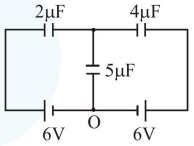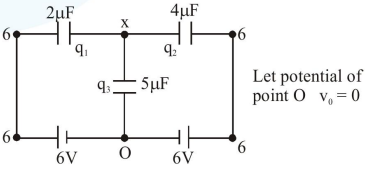# In the circuit shown, charge on theQuestion:

In the circuit shown, charge on the $5 \mu \mathrm{F}$ capacitor is :1. $5.45 \mu \mathrm{C}$

2. $16.36 \mu \mathrm{C}$

3. $10.90 \mu \mathrm{C}$

4. $18.00 \mu \mathrm{C}$

Correct Option: 2,

Solution:Now, using junction analysis

We can say, $\quad q_{1}+q_{2}+q_{3}=0$

$2(x-6)+4(x-6)+5(x)=0$

$\mathrm{x}=\frac{36}{11} \quad \mathrm{q}_{3}=\frac{36(5)}{11}=\frac{180}{11}$

$\mathrm{q}_{3}=16.36 \mu \mathrm{C}$